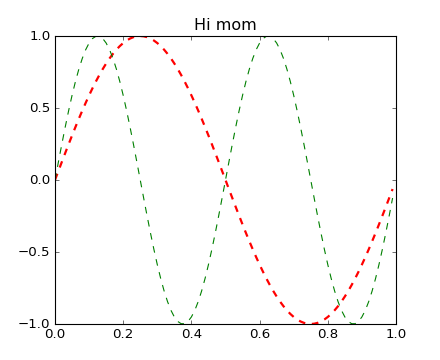## Matplotlib 2.0.0rc2 is available

Install the release candidate now!Travis-CI:# pylab_examples example code: set_and_get.py¶```"""

The pyplot interface allows you to use setp and getp to set and get
object properties, as well as to do introspection on the object

set:
To set the linestyle of a line to be dashed, you can do

>>> line, = plt.plot([1,2,3])
>>> plt.setp(line, linestyle='--')

If you want to know the valid types of arguments, you can provide the
name of the property you want to set without a value

>>> plt.setp(line, 'linestyle')
linestyle: [ '-' | '--' | '-.' | ':' | 'steps' | 'None' ]

If you want to see all the properties that can be set, and their
possible values, you can do

>>> plt.setp(line)

set operates on a single instance or a list of instances.  If you are
in query mode introspecting the possible values, only the first
instance in the sequence is used.  When actually setting values, all
the instances will be set.  e.g., suppose you have a list of two lines,
the following will make both lines thicker and red

>>> x = np.arange(0,1.0,0.01)
>>> y1 = np.sin(2*np.pi*x)
>>> y2 = np.sin(4*np.pi*x)
>>> lines = plt.plot(x, y1, x, y2)
>>> plt.setp(lines, linewidth=2, color='r')

get:

get returns the value of a given attribute.  You can use get to query
the value of a single attribute

>>> plt.getp(line, 'linewidth')
0.5

or all the attribute/value pairs

>>> plt.getp(line)
aa = True
alpha = 1.0
antialiased = True
c = b
clip_on = True
color = b
... long listing skipped ...

Aliases:

To reduce keystrokes in interactive mode, a number of properties
have short aliases, e.g., 'lw' for 'linewidth' and 'mec' for
'markeredgecolor'.  When calling set or get in introspection mode,
these properties will be listed as 'fullname or aliasname', as in

"""

from __future__ import print_function

import matplotlib.pyplot as plt
import numpy as np

x = np.arange(0, 1.0, 0.01)
y1 = np.sin(2*np.pi*x)
y2 = np.sin(4*np.pi*x)
lines = plt.plot(x, y1, x, y2)
l1, l2 = lines
plt.setp(lines, linestyle='--')       # set both to dashed
plt.setp(l1, linewidth=2, color='r')  # line1 is thick and red
plt.setp(l2, linewidth=1, color='g')  # line2 is thicker and green

print('Line setters')
plt.setp(l1)
print('Line getters')
plt.getp(l1)

print('Rectangle setters')
plt.setp(plt.gca().patch)
print('Rectangle getters')
plt.getp(plt.gca().patch)

t = plt.title('Hi mom')
print('Text setters')
plt.setp(t)
print('Text getters')
plt.getp(t)

plt.show()
```

Keywords: python, matplotlib, pylab, example, codex (see Search examples)